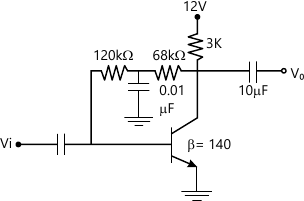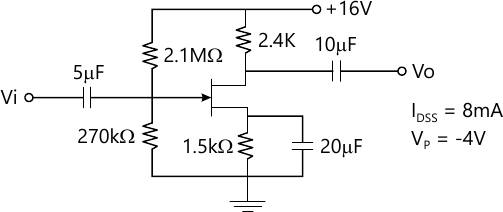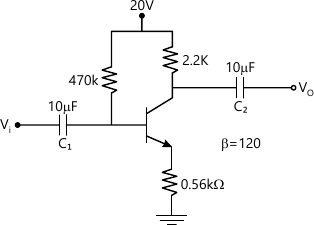MORE IN Analog Electronics - 1
MU Electronics and Telecom Engineering (Semester 3)
Analog Electronics - 1
May 2015
Total marks: --
Total time: --
INSTRUCTIONS
(1) Assume appropriate data and state your reasons
(2) Marks are given to the right of every question
(3) Draw neat diagrams wherever necessary

1 (a) Compare clipper and clamper circuit.
5 M
1 (b) Explain Barkhausen criteria for sustained oscillations.
5 M
1 (c) Compare Depletion and Enhancement type MOSFET.
5 M
1 (d) Transistor is a current controlled device while FET is a voltage controlled device. Justify.
5 M

2 (a) Define Stability factor. Derive the equation for stability factor. State which biasing technique is more stable. Justify your answer.
10 M
2 (b) For a NPN transistor in CE mode voltage divider bias configuration determine VC and VB-. Given VCC=+20V, VEE=-20V, R1=8.2 KkΩ, R2=2.2KΩ, RC=2.7 KΩ, RE=1.8 KΩ, C1=C2=10 7mu;F and β=120.
10 M

3 (a) Derive the equations for Av, Ai, Ri and Ro for a NPN transistor in CE mode voltage divider bias configuration with RE unbypassed.
10 M
3 (b) For the network given below determine Zi, Zo and Av.10 M

4 (a) Explain the basic operation and characteristics of n-channel enhancement type of MOSFET.
10 M
4 (b) Draw a neat circuit diagram of wien bridge oscillator and derive an expression for its output frequency,
10 M

5 (a) Determine IDQ, VGSQ VD & VS for the network given below:10 M
5 (b) Determine Zi Zo & Av for the circuit given below:10 M

Write short note on any four:
6 (a) Biasing of JFET for Zero temperature drift.
5 M
6 (b) Energy band diagram of MOS capacitor.
5 M
6 (c) Small signal equivalent circuit of CC amplifier.
5 M
6 (d) Crystal oscillator.
5 M
6 (e) DC load line & significance of Q point.
5 M

More question papers from Analog Electronics - 1